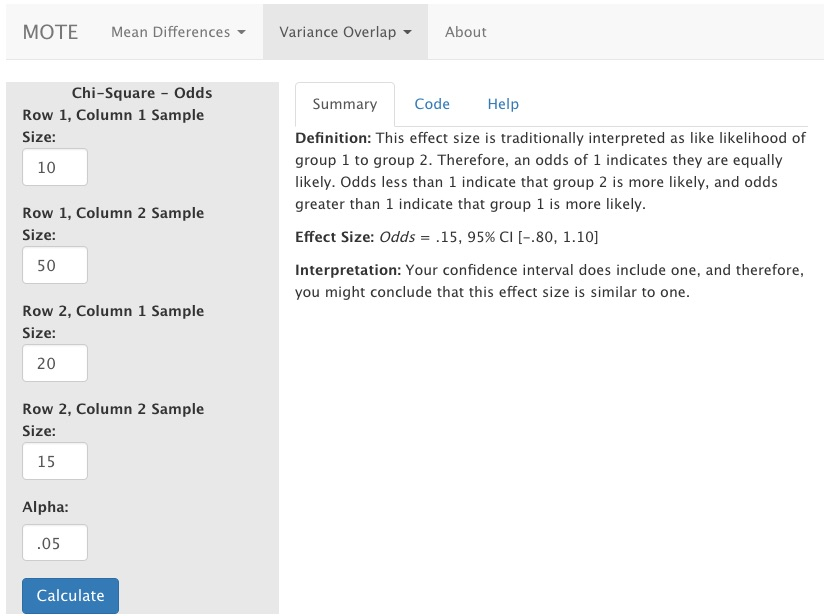# Description

This function displays odds ratios and their normal confidence intervals. This statistic is calculated as (level 1.1/level 1.2) / (level 2.1/level 2.2), which can be considered the odds of level 1.1 given level1 overall versus level2.1 given level2 overall.

The formula for odds is: $$\frac{(N_{11}/N_{12})}{(N_{21}/N_{22})}$$

# R Function

odds(n11, n12, n21, n22, a)

# Arguments

• n11 = sample size for level 1.1
• n12 = sample size for level 1.2
• n21 = sample size for level 2.1
• n22 = sample size for level 2.2
• a = significance level

# Example

A health psychologist was interested in the rates of anxiety in first generation and regular college students. They polled campus and found the following data:

First Generation Regular
Low Anxiety 10 50
High Anxiety 20 15

# Function in R:

odds(n11 = 10, n12 = 50, n21 = 20, n22 = 15, a = 0.05)

# MOTE

## Screenshot## Effect Size:

This effect size is traditionally interpreted as like likelihood of group 1 to group 2. Therefore, an odds of 1 indicates they are equally likely. Odds less than 1 indicate that group 2 is more likely, and odds greater than 1 indicate that group 1 is more likely.

Odds = .15, 95% CI [-.80, 1.10]

## Interpretation:

Your confidence interval does not include one, and therefore, you might conclude that this effect size is different from one.

Not applicable.

Not applicable.

Not applicable.